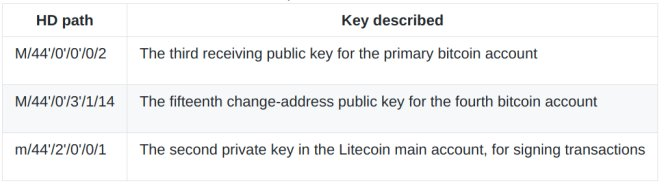Hierarchical Deterministic Wallets(BIP32)

Oveview
For example, when operating a payment system of a Bitcoin at EC site (site for selling and buying goods on the net), the server of EC site does not access the private key for safety, and It is necessary to generate payment address for settlement.
But, in the case of Bitcoin core wallet, 100 keys are cached in the pool of reserved keys by default, so this wallet can not be operated simultaneously in multiple systems. Also Bitcoin core can use the encryption function to protect the secret key in a way that does not share the password, but can not generate the public key.

HD wallet is a method of generating a private key from one seed (random number) and managing it using a hierarchical structure. Back up the seed only once, it can restore the key and it will be easier to manage. It can safely generate the public key without exposing the private key, generate a payment address for each remittance, and manage those balances as well. In HD Wallet, it change Bitcoin address every receive / sending, it is the basic design, protecting security or privacy at the same time while maintaining the convenience of backpack.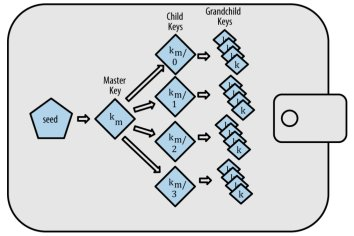Generate HD wallets from seed
1.Secure pseudo-random number generator generate to Root Seed(128-256 bits)

2.Root Seed(512bit) is generated using PBKDF2(key extended function)from seed(128-256bits)

3.Hash value is calculated from Root Seed by using HMAC-SHA512

4.Left half of hash become 'Master private key',and right half of hash become 'Master chain code'

5.The generate method of master public key is exactly the same method which was introduced in past articles(using ECDSA to private key)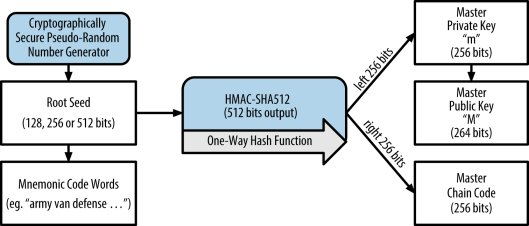Master private key & Master chain code

```def bip32_master_key(seed):
I = hmac.new(bytes("Bitcoin seed", 'utf-8'), seed, hashlib.sha512).digest()

depth = 0
fingerprint = b'\x00\x00\x00\x00'
i = b'\x00\x00\x00\x00'
chaincode = encode(decode(I[32:], 256), 256, 32)
keydata = b'\x00'+(I[:32]+b'\x01')[:-1]

bindata = vbytes + bytes([depth % 256]) + fingerprint + i + chaincode + keydata
string=bindata+hashlib.sha256(hashlib.sha256(bindata).digest()).digest()[:4]
return encode(decode(string, 256), 58, 0)

bip32_masterprivatekey = bip32_master_key(privatekey)
```

def bip32_master_key(seed):
→"seed" use Root seed(512bit)

I = hmac.new(bytes("Bitcoin seed", 'utf-8'), seed, hashlib.sha512).digest()
→Calculate of Hmac use Python module　※showing 1 to 3 of above procedure

chaincode = encode(decode(I[32:], 256), 256, 32)
→Generate master chain code

bindata = vbytes + bytes([depth % 256]) + fingerprint + i + chaincode + keydata
→Encode by Base58chack for sum ① to ⑥
①vbytes(Prefix of private key defined by BIP32)
②depth(Derived path depth)
③fingerprin(Connection with parents)
④i(Key index)
⑤chaincode(Master chaincode)
⑥keydata(Master private key)
※The value is 0 for initial state about ② to ④

string=bindata+hashlib.sha256(hashlib.sha256(bindata).digest()).digest()
→Behind bin data add checksum generate to Master private key.

Master public key

```def bip32_master_public_key(rawtuple):
dbin = encode(decode(rawtuple, 58), 256, 0)

vbytes = dbin[0:4]
depth = dbin
fingerprint = dbin[5:9]
i = dbin[9:13]
chaincode = dbin[13:45]
key = dbin[46:78]+b'\x01'
newvbytes = b'\x04\x88\xB2\x1E'

pub = fast_multiply(G, decode(key[:32], 256))
keydata = bytes([2+(pub % 2)]) + encode(pub, 256, 32)

bindata = newvbytes + bytes([depth % 256]) + fingerprint + i + chaincode + keydata
return encode(decode((bindata+hashlib.sha256(hashlib.sha256(bindata).digest()).digest()[:4]), 256), 58, 0)

bip32_masterpublickey = bip32_master_public_key(bip32_masterprivatekey)
```

dbin = encode(decode(rawtuple, 58), 256, 0)
→Convert hex to byte data of 256-hex,It contains the following information.
①dbin[0:4]:vbytes(4byte)
②dbin:depth(1byte)
③dbin[5:9]:fingerprint(4byte)
④dbin[9:13]:i(4byte)
⑤dbin[13:45]:chain code(33byte)
⑥dbin[46:78]:key(33byte)

pub = fast_multiply(G, privkey)
→Use ECDSA to private key

keydata = bytes([2+(pub % 2)]) + encode(pub, 256, 32)
→Generate compressed public key

bindata = newvbytes + bytes([depth % 256]) + fingerprint + i + chaincode + keydata
→Add edited data from ① to ⑥

encode(decode((bindata+hashlib.sha256(hashlib.sha256(bindata)...
→It encode by Base58chack

```def pubkey_to_address(pubkey):
dbin = encode(decode(pubkey, 58), 256, 0)
key = dbin[45:78]
return bin_to_b58check(bin_hash160(key))

```

Generate process of address is exactly the same previous method.
As noteworthy,the key data Included in Master public key is not start from 46-byte but 45-byte.

Derivation of child private key
Deterministic Wallets created child key from parent key using "Child key derivation (CKD)" functions.

1.Parent Public Key(264-bit) is generated from Parent Private Key(256-bit: uncompressed key)

2.Hash the　below combination in HMAC-SHA 512
Parent Public Key(264-bit), Parent Chain Code(256-bit), Index number(32-bit)

3.Hashed value of left half is child private key,and other half is child chain code(256-bits)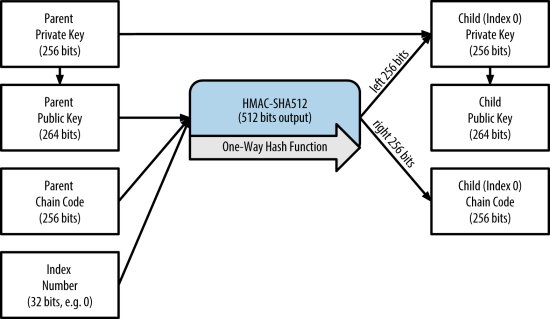```def bip32_ckd_prv(data, i = int(i)):
dbin = encode(decode(data, 58), 256, 0)
vbytes = dbin[0:4]
depth = dbin + 1
chaincode = dbin[13:45]
key = dbin[46:78]+b'\x01'
fingerprint = bin_hash160(privkey_to_pubkey(key))[:4]
keyindex = encode(i, 256, 4)
I = hmac.new(chaincode, privkey_to_pubkey(key)+ encode(i, 256, 4), hashlib.sha512).digest()
chaincode = encode(decode(I[32:], 256), 256, 32)
newkey = encode((decode((I[:32]+B'\x01')[:32], 256) + decode(key[:32], 256)) % N, 256, 32)+b'\x01'
newkey = b'\x00'+newkey[:-1]

bindata = vbytes + bytes([depth % 256]) + fingerprint + keyindex + chaincode + newkey
return encode(decode(bindata+ hashlib.sha256(hashlib.sha256(bindata).digest()).digest()[:4], 256), 58, 0)

print(bip32_ckd_prv(bip32_masterprivatekey, 1))
```

depth = dbin + 1
→The depth of the derived path is deepened only one

key = dbin[46:78]+b'\x01'
→Private key add suffix 0x01 when WIF-compressed format

I = hmac.new(chaincode, privkey_to_pubkey(key)...
→HMAC hashed to parent public key and parent chain Code and key index

newkey = encode((decode((I[:32]+B'\x01')[:32]...
→Child private key is deriveｄ from add front half of hash to parente private key

bindata = vbytes + bytes([depth % 256])...
→prefix + depth + fingerprint + i + chaincode + keydata

Derivation of child public key
Hierarchical Deterministic Wallets have very useful characteristics that can generate child public key from a parent public key without using a private key.

1.Hash the below combination in HMAC-SHA 512
Parent Public Key(264-bit), Parent Chain Code(256-bit), Index number(32-bit)

2.Hashed value of left half is child public key,and other half is child chain code(256-bits)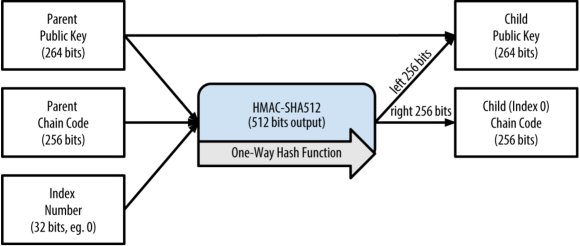```def bip32_ckd(data, i = int(i)):
dbin = encode(decode(data, 58), 256, 0)
vbytes = dbin[0:4]
depth = dbin + 1
key = dbin[45:78]
fingerprint = bin_hash160(key)[:4]
i = encode(i, 256, 4)
chaincode = dbin[13:45]
I = hmac.new(chaincode, key + i, hashlib.sha512).digest()
chaincode = encode(decode(I[32:], 256), 256, 32)
pub = decode_pubkey(privkey_to_pubkey(I[:32]))
keydata = add_pubkeys(bytes([2+((pub) % 2)]) + encode((pub), 256, 32), key)

bindata = vbytes + bytes([depth % 256]) + fingerprint + i + chaincode + keydata
string = bindata+hashlib.sha256(hashlib.sha256(bindata).digest()).digest()[:4]
return encode(decode(string, 256), 58, 0)

ckd_publickey  = bip32_ckd(bip32_masterpublickey, 1)
```

pub = decode_pubkey(privkey_to_pubkey(I[:32]))
→Compressed public key is derived, it use to calculate x and y coordinate

keydata = add_pubkeys(bytes([2+((pub) % 2)]) + encode((pub), 256, 32), key)
→On jacobian coordinate,Add x coordinate and prefix(2 or 3) to Master public key

Add suffix(first 4byte) of double hashed bindata to bindata,it encode by chack58encode.

hardened derivation
Hardened derivation pubkey is generated child chain code using parent privkey instead of parent pubkey,destroy relationship between pubkey and child chain code.
Nomal derivation fanction use index for 2 to 2^32-1(0x80000000 to 0xFFFFFFFF),Hardened derivation function use 0 to 2^31 -1(0x0 to 0x7FFFFFFF).

Why destory relationship?
Method of generating branch of pubkey from extended pubkey has potential　risk.
Because extended pubkey is included in chain code,if single child privkey leak out become all child privkey leak out.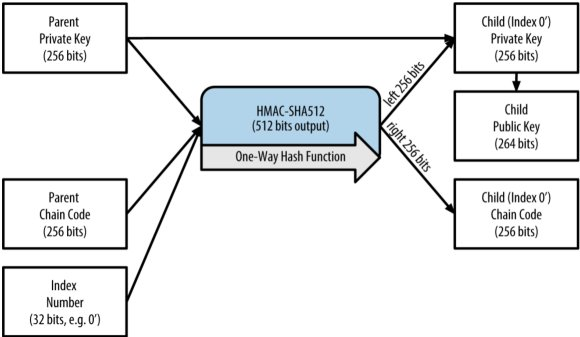Extended key
Extended private key(parent privkey + chain code)
It possible to generate child privkey and child pubkey.
Expanded privkey can create complete branch root.
Prefix become "xprv"

`xprv9tyUQV64JT5qs3RSTJkXCWKMyUgoQp7F3hA1xzG6...`

Extended public key(parent pubkey + chain code)
It possible to generate child pubkey.
Expand privkey can create branch of pubkey only.
Prefix become "xpub"

`xpub67xpozcx8pe95XVuZLHXZeG6XWXHpGq6Qv5cmNfi...`

HD wallet path
The key of HD wallet is determined uniquely using "pass".
Tree hierarchies are separated by slashes.
" ' " indicates that hardened derivation is used.
" m " shows that is generated private key from master privkey.
" M " is generated public key from master public key.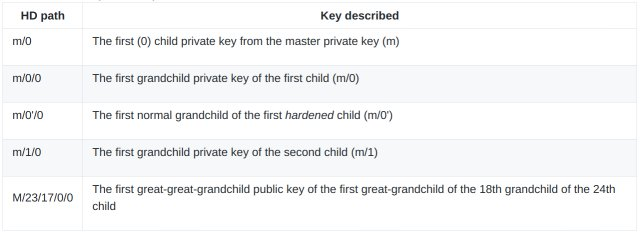HD wallet tree
Parent extended key can generate child key of 4 billion,
however data move to other wallet, takes an enormous amount of time.
Two BIP0043 and BIP0044 proposing for this problem solution.

BIP0043/BIP0044
BIP0043 proposed the first level of BIP32 tree structure to be used as "purpose".
By extending this BIP, BIP 0044 defines the number "44 '" representing t "purpose" based on BIP 0043.
The ｆirst layer is " m / 44 ' / " and "44” show number of purpose.
It means that wallet adapt construction of BIP0044.
BIP0044 aim to improve Wallet's compatibility by unifying rules.

`m / purpose' / coin_type' / account' / change / address_index`

purpose :Become "44" at all time

coin_type' :Kinds of coin(if Bitcoin"m/44'/0' " or Litecoin's "m/44'/2'")

account' :Multiple accounts number(i)